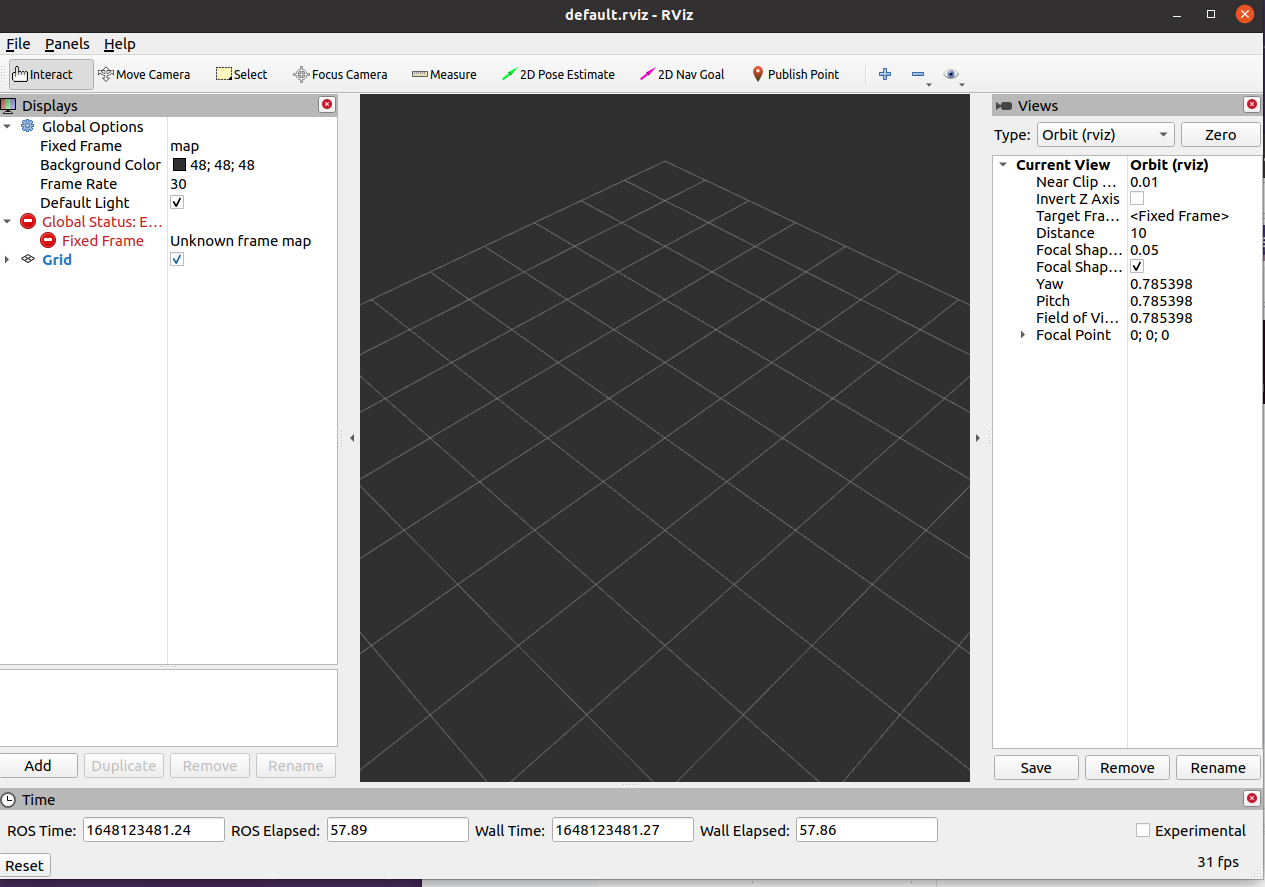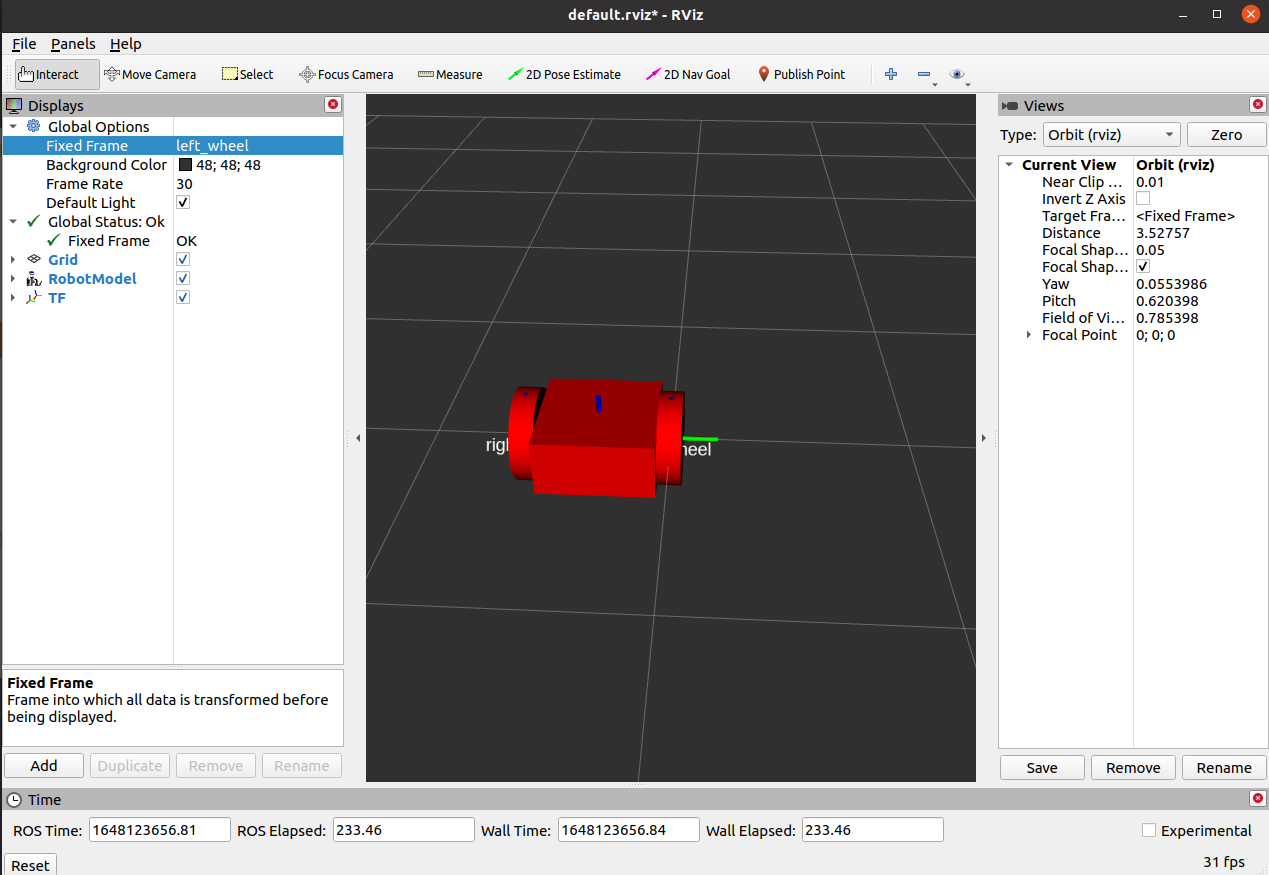# ros学习之机器人构建以及rviz可视化机器人

1、ros相关的操作语句，例如构建工作区间，创建文件夹以及文件。

2、XML语言。

3. 根据需要，修改URDF文件
4. 建立launch启动文件

1、首先在自己的工作区间内新建一个ros软件包，我的工作区间是catkin_ws（相关文件的构建可以去维基中文版学习，见如下链接：cn/ROS/Tutorials - ROS Wiki）。

cd ~/catkin_ws/src
catkin_create_pkg ros_robotics

2、接着对这个软件包进行编译

cd(该命令回到始目录)

cd ~/catking_ws（进入工作区间）
catkin_make（进行编译）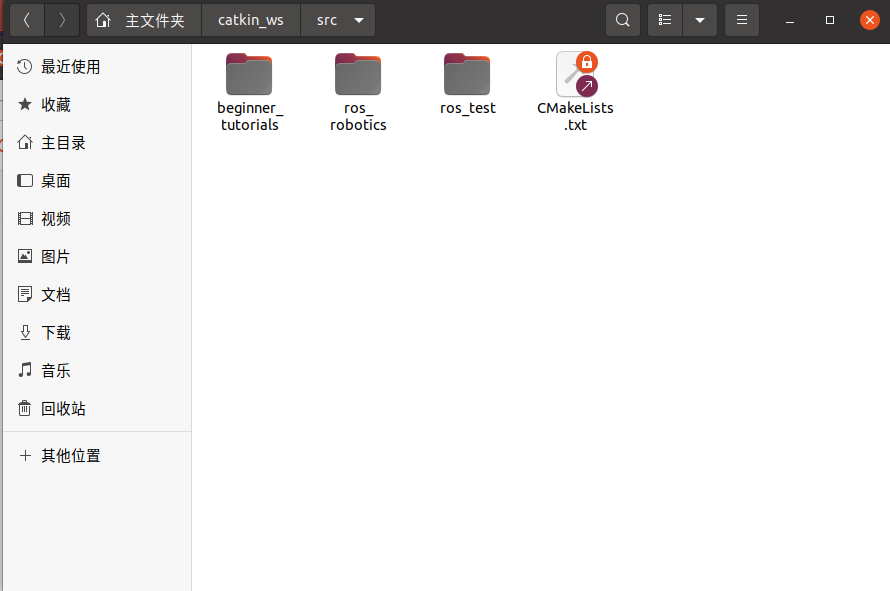另外两个ros软件包是实现其他功能的包，其构建方法类似，修改下文件名即可。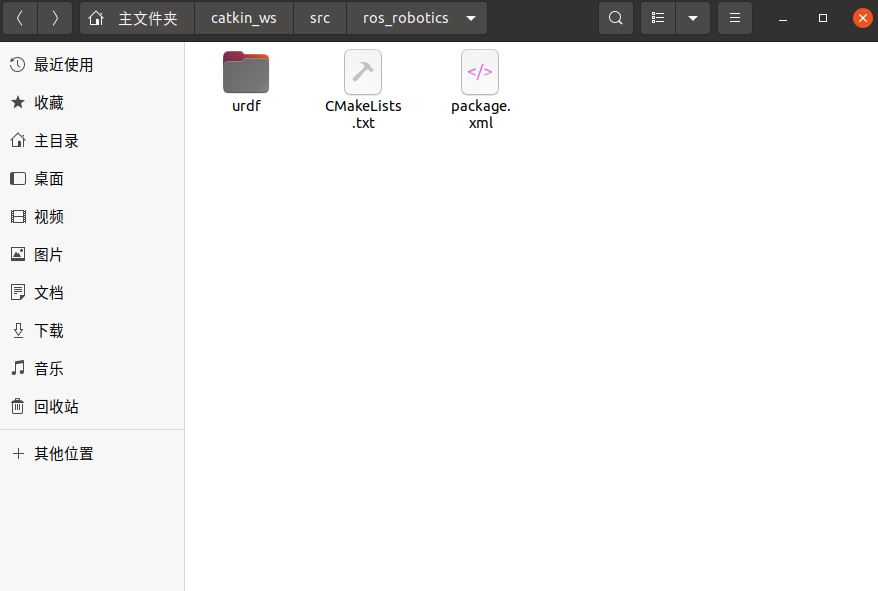在urdf文件中新建一个.urdf文件（一定要先定位到该文件夹）：

cd ~catkin_ws/src/ros_robotics/urdf

touch dd_robot.urdf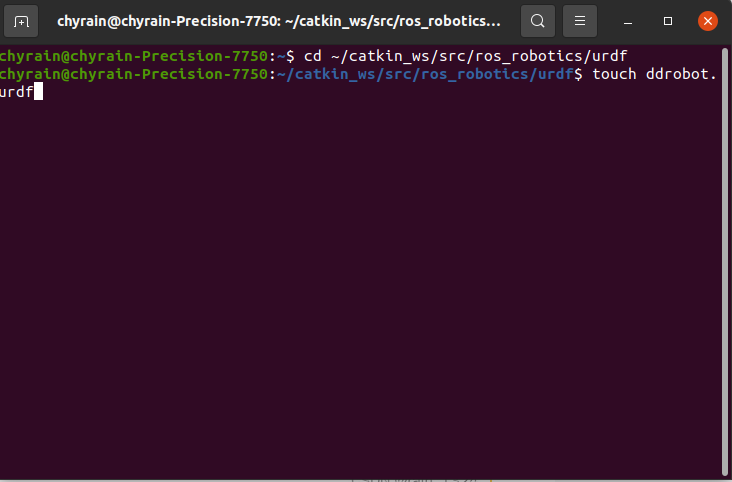然后可以看到文件中出现一个dd_robot.urdf文件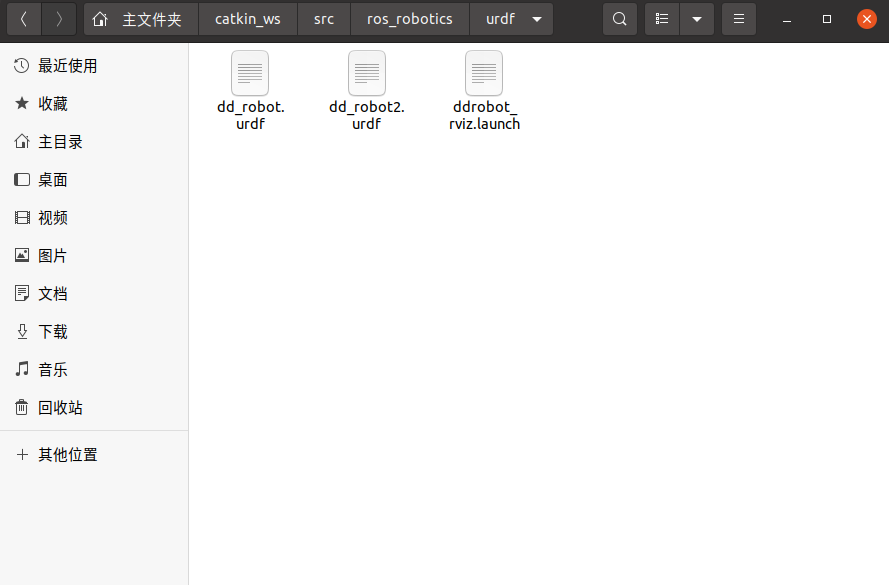双击打开.urdf文件，然后复制如下代码并保存：

<robot name="dd_robot">
<visual>
<origin xyz="0 0 0" rpy="0 0 0"/>
<geometry>
<box size="0.5 0.5 0.25"/>
</geometry>
</visual>

<!-- Right Wheel -->
<visual>
<origin xyz="0 0 0" rpy="1.570795 0 0"/>
<geometry>
<cylinder length="0.1"
</geometry>
</visual>
<joint name="joint_right_wheel" type="continuous">
<origin xyz="0 -0.30 0" rpy="0 0 0"/>
<axis xyz="0 1 0"/>
</joint>
<!-- Left Wheel -->
<visual>
<origin xyz="0 0 0"
rpy="1.570795 0 0"/>
<geometry>
<cylinder length="0.1"
</geometry>
</visual>
<joint name="joint_left_wheel"
type="continuous">
<origin xyz="0 0.30 0" rpy="0 0 0"/>
<axis xyz="0 1 0"/>
</joint>
</robot>

joint组件描述了连接的运动学以及动态（包括连接的连接杆、关节类型、旋转轴、摩擦力和阻尼的合力等）

3、完成上述操作后，我们需要一个.launch文件来将其在rviz中可视化，同理要先进到该文件的路径中：

cd ~catkin_ws/src/ros_robotics/urdf

touch ddrobot_rviz.launch

<launch>
<arg name="model" />
<arg name="gui" default="False" />
<!-- set these parameters on Parameter Server -->
<param name="robot_description" textfile="\$(find
ros_robotics)/urdf/\$(arg model)" />
<param name="use_gui" value="\$(arg gui)"/>
<!-- Start 3 nodes: joint_state_publisher,
robot_state_publisher and rviz -->
<node name="joint_state_publisher"
pkg="joint_state_publisher" type="joint_state_publisher" />
<node name="robot_state_publisher"
pkg="robot_state_publisher" type="robot_state_publisher" />
<node name="rviz" pkg="rviz" type="rviz" args="-d \$(find
ros_robotics)/urdf.rviz" required="true" />
<!-- (required = "true") if rviz dies, entire roslaunch will be
killed -->
</launch>

4、打开终端，首先进入到工作区间内，然后source一下：

cd ~/catkin_ws

source devel/setup.bash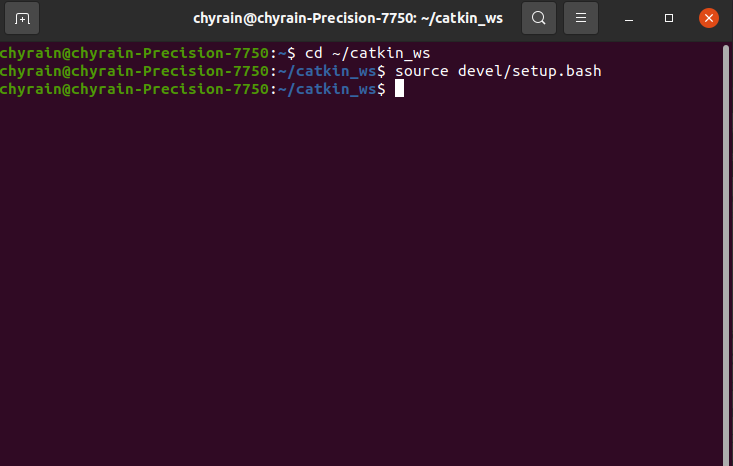roslaunch ros_robotics ddrobot_rviz.launch model:=dd_robot.urdf